# 14-240/Classnotes for Monday September 22

Polar coordinates:

•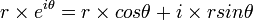$r \times e^{i\theta} = r \times cos\theta + i \times rsin\theta$
•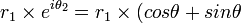$r_1 \times e^{i\theta_2} = r_1 \times (cos\theta + sin\theta$

The Fundamantal Theorem of Algebra: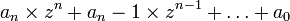$a_n \times z^{n} + a_n-1 \times z^{n-1} + \dots + a_0$ where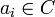$a_i \in C$and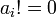$a_i != 0$ has a soluion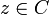$z \in C$ In particular,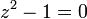$z^{2} - 1 = 0$ has a solution.

• Forces can multiple by a "scalar"(number).

No "multiplication" of forces.

Definition of Vector Space: A "Vector Space" over a field F is a set V with a special element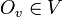$O_v \in V$ and two binary operations:

•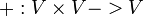$+ : V \times V -> V$
•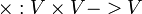$\times : V \times V -> V$

s.t.

•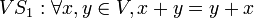$VS_1 : \forall x, y \in V, x + y = y + x$.
•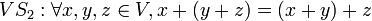$VS_2 : \forall x, y, z \in V, x + (y + z) = (x + y) + z$.
•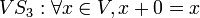$VS_3 : \forall x \in V, x + 0 = x$.
•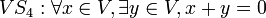$VS_4 : \forall x \in V, \exists y \in V, x + y = 0$.
•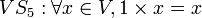$VS_5 : \forall x \in V, 1 \times x = x$.
•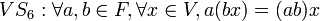$VS_6 : \forall a, b \in F, \forall x \in V, a(bx) = (ab)x$.
•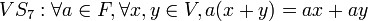$VS_7 : \forall a \in F, \forall x, y \in V, a(x + y) = ax + ay$.
•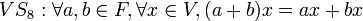$VS_8 : \forall a, b \in F, \forall x \in V, (a + b)x = ax + bx$.